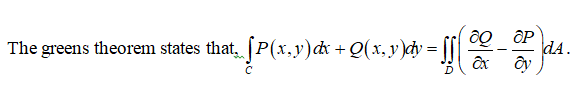# Use Green's Theorem to evaluate the integral of F · dr. over C (Check the orientation of the curve before applying the theorem.)F(x, y) = (y2 cos(x), x2 + 2y sin(x)) C is the triangle from (0, 0) to (1, 3) to (1, 0) to (0, 0)

Question
8 views

Use Green's Theorem to evaluate

the integral of F · dr. over C

(Check the orientation of the curve before applying the theorem.)

F(x, y) =
(y2 cos(x), x2 + 2y sin(x))

C is the triangle from (0, 0) to (1, 3) to (1, 0) to (0, 0)
check_circle

Step 1Step 2

Sketch the triangle as,

Step 3...

### Want to see the full answer?

See Solution

#### Want to see this answer and more?

Solutions are written by subject experts who are available 24/7. Questions are typically answered within 1 hour.*

See Solution
*Response times may vary by subject and question.
Tagged in

### Math